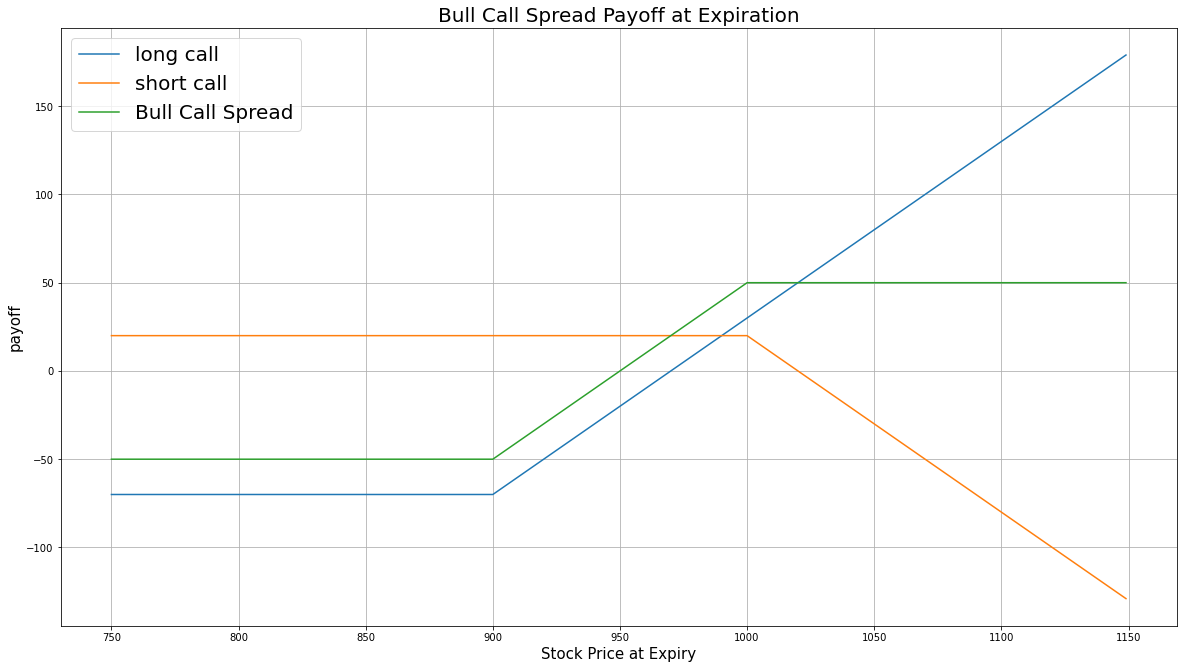# Option Strategies

### Introduction

Bull call spread, also known as long call spread, consists of buying an ITM call and selling an OTM call. Both calls have the same underlying Equity and the same expiration date. The OTM call serves as a hedge for the ITM call. The bull call spread profits from a rise in underlying asset price.

### Implementation

1. In the Initialize method, set the start date, end date, cash, and Option universe.
2. private Symbol _symbol;

public override void Initialize()
{
SetStartDate(2017, 2, 1);
SetEndDate(2017, 3, 5);
SetCash(500000);

_symbol = option.Symbol;
option.SetFilter(universe => universe.IncludeWeeklys()
.Strikes(-15, 15)
.Expiration(TimeSpan.FromDays(0), TimeSpan.FromDays(31)));
}
def Initialize(self) -> None:
self.SetStartDate(2017, 2, 1)
self.SetEndDate(2017, 3, 5)
self.SetCash(500000)

self.symbol = option.Symbol
option.SetFilter(self.UniverseFunc)

def UniverseFunc(self, universe: OptionFilterUniverse) -> OptionFilterUniverse:
return universe.IncludeWeeklys().Strikes(-15, 15).Expiration(timedelta(0), timedelta(31))
3. In the OnData method, select the expiration and strikes of the contracts in the strategy legs.
4. public override void OnData(Slice slice)
{
if (Portfolio.Invested) return;

// Get the OptionChain
var chain = slice.OptionChains.get(_symbol, null);
if (chain.Count() == 0) return;

// Get the furthest expiration date of the contracts
var expiry = chain.OrderByDescending(x => x.Expiry).First().Expiry;

// Select the call Option contracts with the furthest expiry
var calls = chain.Where(x => x.Expiry == expiry && x.Right == OptionRight.Call);
if (calls.Count() == 0) return;

// Select the ITM and OTM contract strikes from the remaining contracts
var putStrikes = calls.Select(x => x.Strike).OrderBy(x => x);
var itmStrike = putStrikes.First();
var otmStrike = putStrikes.Last();
def OnData(self, slice: Slice) -> None:
if self.Portfolio.Invested: return

# Get the OptionChain
chain = slice.OptionChains.get(self.symbol, None)
if not chain: return

# Get the furthest expiration date of the contracts
expiry = sorted(chain, key = lambda x: x.Expiry, reverse=True).Expiry

# Select the call Option contracts with the furthest expiry
calls = [i for i in chain if i.Expiry == expiry and i.Right == OptionRight.Call]
if len(calls) == 0: return

# Select the ITM and OTM contract strike prices from the remaining contracts
call_strikes = sorted([x.Strike for x in calls])
itm_strike = call_strikes
otm_strike = call_strikes[-1]
5. In the OnData method, call the OptionStrategies.BullCallSpread method and then submit the order.
6. var optionStrategy = OptionStrategies.BullCallSpread(_symbol, itmStrike, otmStrike, expiry);
Buy(optionStrategy, 1);
option_strategy = OptionStrategies.BullCallSpread(self.symbol, itm_strike, otm_strike, expiry)
self.Buy(option_strategy, 1)

Option strategies synchronously execute by default. To asynchronously execute Option strategies, set the asynchronous argument to Falsefalse. You can also provide a tag and order properties to the Buy method.

Buy(optionStrategy, quantity, asynchronous, tag, orderProperties);

self.Buy(option_strategy, quantity, asynchronous, tag, order_properties)


### Strategy Payoff

The bull call spread is a limited-reward-limited-risk strategy. The payoff is

$$\begin{array}{rcll} C^{OTM}_T & = & (S_T - K^{OTM})^{+}\\ C^{ITM}_T & = & (S_T - K^{ITM})^{+}\\ P_T & = & (C^{ITM}_T - C^{OTM}_T + C^{OTM}_0 - C^{ITM}_0)\times m - fee\\ \end{array}$$ $$\begin{array}{rcll} \textrm{where} & C^{OTM}_T & = & \textrm{OTM call value at time T}\\ & C^{ITM}_T & = & \textrm{ITM call value at time T}\\ & S_T & = & \textrm{Underlying asset price at time T}\\ & K^{OTM} & = & \textrm{OTM call strike price}\\ & K^{ITM} & = & \textrm{ITM call strike price}\\ & P_T & = & \textrm{Payout total at time T}\\ & C^{ITM}_0 & = & \textrm{ITM call value at position opening (debit paid)}\\ & C^{OTM}_0 & = & \textrm{OTM call value at position opening (credit received)}\\ & m & = & \textrm{Contract multiplier}\\ & T & = & \textrm{Time of expiration} \end{array}$$

The following chart shows the payoff at expiration:The maximum profit is $K^{OTM} - K^{ITM} + C^{OTM}_0 - C^{ITM}_0$. If the underlying price increases to exceed both strikes at expiration, both calls are worth $(S_T - K)$ at expiration.

The maximum loss is the net debit you paid to open the position, $C^{OTM}_0 - C^{ITM}_0$.

If the Option is American Option, there is a risk of early assignment on the sold contract.

### Example

The following table shows the price details of the assets in the algorithm:

AssetPrice ($)Strike ($)
OTM call3.00835.00
ITM call41.00767.50
Underlying Equity at expiration829.08-

Therefore, the payoff is

$$\begin{array}{rcll} C^{OTM}_T & = & (S_T - K^{OTM})^{+}\\ & = & (829.08-835.00)^{+}\\ & = & 0\\ C^{ITM}_T & = & (S_T - K^{ITM})^{+}\\ & = & (829.08-767.50)^{+}\\ & = & 61.58\\ P_T & = & (C^{ITM}_T - C^{OTM}_T + C^{OTM}_0 - C^{ITM}_0)\times m - fee\\ & = & (61.58-0+3.00-41.00)\times100-1.00\times2\\ & = & 2356\\ \end{array}$$

So, the strategy profits \$2,356.

The following algorithm implements a bull call spread strategy:

You can also see our Videos. You can also get in touch with us via Discord.